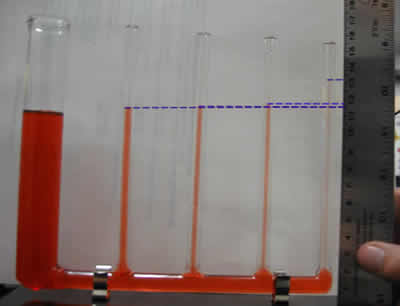## Capillary Equilibrium

Author(s): Christopher Chui and Jim Mauch
Demonstration Equipment - Teacher's Guide
SED 695B; Fall 2005

Principles illustrated:

• Capillary action
• Equilibrium
• Balanced forcesHigh school:

1. Newton's laws predict the motion of most objects. As a basis for understanding this concept: Students know that when forces are balanced, no acceleration occurs; thus an object continues to move at a constant speed or stays at rest (Newton's first law).
Students know the relationship between the universal law of gravitation and the effect of gravity on an object at the surface of the Earth.

Middle school

Unbalanced forces cause changes in velocity. As a basis for understanding this concept:
Students know when an object is subject to two or more forces at once, the result is the cumulative effect of all the forces.
Students know how to identify separately the two or more forces that are acting on a single static object, including gravity, elastic forces due to tension or compression in matter, and friction.

Materials
Explanation of principles involved

Capillary equilibrium tube

50ml of water

food coloring

• Normally, we would expect the height of water in a series of connected tubes to be equal, since gravitational pull and air pressure are acting on them equally.
• In this case, the tubes are so small that capillary action plays a role.
• The tendency for water molecules to be attracted to other substances (adhesive force) as well as surface tension pull the water column up until these forces are matched by the weight of the water lifted and its cohesive force (the force which draws molecules in a liquid together.
• In a narrower tube, the adhesive force has a greater effect than the force of gravity, so the water column climbs until equilibrium is reached.
• The action of the same forces is what causes the formation of a meniscus in a graduated cylinder, test tube or simply a glass of water.
• A liquid like mercury has a very low adhesive force. In fact, it tends to repel from other substances. The result of this is the formation of the reverse of a meniscus. The liquid level is lower at the edges than it is in the center.
 COLUMN HEIGHT AND DIAMETER A B C D E Height (cm) 10.1 10.9 10.9 11.1 12.55 Diameter (cm) 1.9 0.4 0.3 0.2 0.1 Radius (cm) 0.95 0.2 0.15 0.1 0.05 h (cm) 0 0.8 0.8 1 2.45 Height (m) 0.101 0.109 0.109 0.111 0.1255 Diameter (m) 0.019 0.004 0.003 0.002 0.001 Radius (m) 0.0095 0.002 0.0015 0.001 0.0005 h (m) 0 0.008 0.008 0.01 0.0245 THEORETICAL PREDICTION OF HEIGHT USING h = (2*y*cos(theta))/(p*r*g) where y = 0.073 N/m theta is 0 degree p = density of water minus the density of air p = 1000 kg/m^3 r = radius of tube g = gravitational acceleration = 9.8 m/sec^2 0.001568206 0.00744898 0.00993197 0.014898 0.029796 Discrepancy Factor 0 1.073972603 0.80547945 0.671233 0.82226 The Discrepancy Factor is now acceptable, due to errors in reading the ruler about the height and diameter of the capillary tubes. Error % -7.397260274 19.4520548 32.87671 17.77397 Conclusion: More careful reading may yield better results.

Procedure:

• Mix a drop or two of food coloring with about 50ml of water
• Ask students to predict how the water levels in the four tubes will compare when water is added
• Pour the water into the large tube
• Students can measure the difference in height of each of the tubes
• Comparisons can be made to other examples of capillary action, for instance, water climbing up a piece of paper that is partially submerged in water.
• A discussion dan be made as to how a tree pumps water all the way up to it's leaves. (The answer is, it doesn't. Capillary action accounts for most of the upward motion of water in the xylem of a tree.)